Next: Worked example 5.6: Driving Up: Conservation of energy Previous: Worked example 5.4: Roller

## Worked example 5.5: Sliding down a plane

Question: A block of mass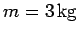starts at rest at a height of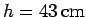on a plane that has an angle of inclination of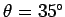with respect to the horizontal. The block slides down the plane, and, upon reaching the bottom, then slides along a horizontal surface. The coefficient of kinetic friction of the block on both surfaces is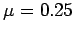. How far does the block slide along the horizontal surface before coming to rest?

Answer: The normal reaction of the plane to the block's weight is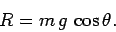Hence, the frictional force acting on the block when it is sliding down the plane isThe change in gravitational potential energy of the block as it slides down the plane is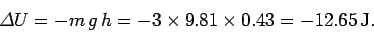The work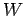done on the block by the frictional force during this process is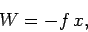where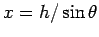is the distance the block slides. The minus sign indicates thatacts in the opposite direction to the displacement of the block. Hence,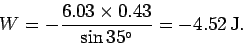Now, by energy conservation, the kinetic energyof the block at the bottom of the plane equals the decrease in the block's potential energy plus the amount of work done on the block: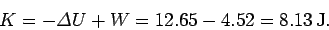The frictional force acting on the block when it slides over the horizontal surface is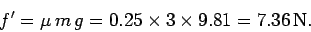The work done on the block as it slides a distanceover this surface is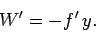By energy conservation, the block comes to rest when the action of the frictional force has drained all of the kinetic energy from the block: i.e., when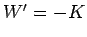. It follows that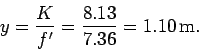Next: Worked example 5.6: Driving Up: Conservation of energy Previous: Worked example 5.4: Roller
Richard Fitzpatrick 2006-02-02